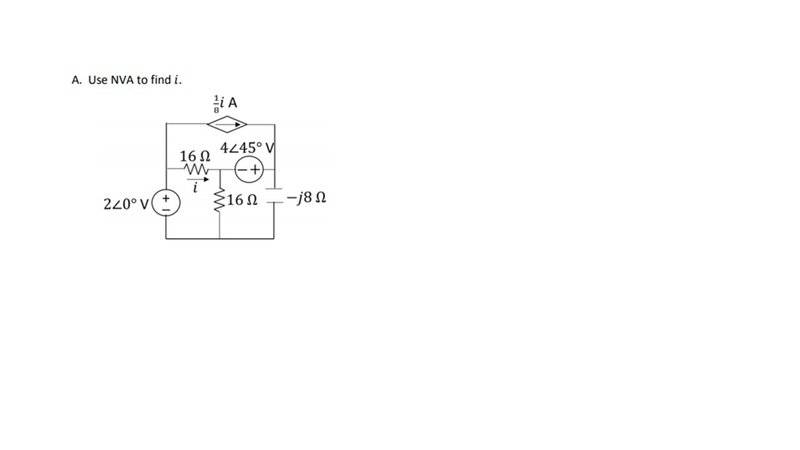# NVA With Complex Numbers

Staff Emeritus

## Homework Statement

Use NVA to solve for ##i##.## The Attempt at a Solution

My nodes are as follows: ##V_1## on the left middle junction, ##V_2## is the junction in the very center, and ##V_3## is the junction on the middle right, just above the capacitor.

I set up ##V_2## and ##V_3## as a supernode and found 3 equations:
##i=\frac{V_1-V_2}{16}##
##16i=V_1-V_2##
##V_2+16i=V_1##

##1). V_2+0V_3+16i=2∠0°##

Supernode:
##-i+\frac{V_2}{16}-\frac{i}{8}+\frac{V_3}{-8j}=0##
##-16i+V_2-2i-\frac{2V_3}{j}=0##

##2.) V_2-\frac{2V_3}{j}-18i=0##

Across the source in the supernode:
##V_3-V_2 = 4∠45°##

##3.) -V_2+V_3+0i=4∠45°##

That's 3 equations and 3 unknowns. Solving for ##i## gives me ##0.486∠-20.45°##, which isn't correct.

#### Attachments

donpacino
Gold Member
To use NVA (also known as nodal analysis) you want to express everything as a bunch of KCLs.

Your equations are using voltage, which is complicating things (KVLs). I personally find it easier to do all current going out or current going in. mixing the two can get confusing.

There are two unknown voltages, v2 and v3. v1 is just 2 volts.

You need one equation (KCL) at your super-node, with the Currents going out (or in)
One equation relating V2 and V3 (you already have that)
One equation relating V2 and i (you already mentioned V2+16i=V1)

Last edited:
donpacino
Gold Member
Ahhh i see what you did, you initially did nodal analysis, but then converted the equations the a solvable format.
disregard my previous post.

Your equations look correct. I got different answers wiht the same equations, it is possible you made a mistake with solving your equations?

Staff Emeritus
Your equations look correct. I got different answers wiht the same equations, it is possible you made a mistake with solving your equations?

It's always possible, but I've put these numbers into my calculator to solve at least a dozen times and I can't find where I might have gone wrong (and it hasn't failed to correctly solve a problem yet. At least when I've entered everything in correctly).

donpacino
Gold Member
What are you getting for your node voltages?

Staff Emeritus
What are you getting for your node voltages?
I'm getting:
##V_2=7.317∠-95.6°##
##V_3=4.929∠-64.61°##
I'm in degrees like the problem tells me to be in.

donpacino
Gold Member
ok, thats different than im getting, are you solving it using a matrix?

the way I solved it, I used X=A^-1 * B

from our system of equations
A= [ 1 0 16 ; 1 0+2j -18 ; -1 1 0 ]

B = [2 0 2.82+2.82j]

Do you have anything different?

Staff Emeritus
I figured it out. I was putting ##16i## instead of ##16## into my calculator for the 1st equation, thinking that ##i## was the imaginary unit instead of ##j##.•I figured it out. I was putting ##16i## instead of ##16## into my calculator for the 1st equation, thinking that ##i## was the imaginary unit instead of ##j##.